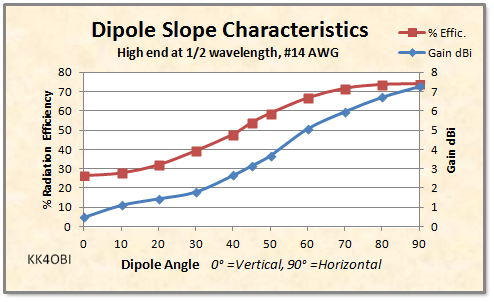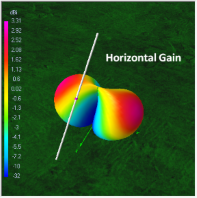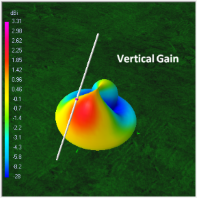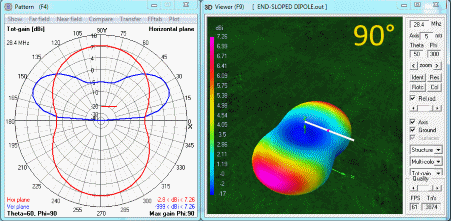This is a discussion about the results of antenna modeling of a half-wave dipole set at various angles of slope.

Center-fed Bent-Dipoles

Horizontal Lateral
Vertical

OCF
EFHW

Slow-Wave
Other Topics

Home
Having only a single vertical support or limited horizontal space might be a problem. Such a problem can be solved by mounting a dipole at a slope.  This is a common antenna configuration in the world of amateur radio referred to as a "Sloper" or more accurately, "Half-Wave Sloper".  This antenna can be angled anywhere in a range from 0° as a Half-Wave Vertical Dipole, to 90° as a flat-top Horizontal Dipole.
To help make a decision about using a sloped dipole "Sloper", you need to know...

What happens as the slope of a dipole changes?

The answers are most quickly found by antenna modeling.  For this study the conditions were set for a center-fed, half-wave resonant wire,  #14 AWG, with the high end fixed at 1/2 wavelength elevation over average "real ground".  Note: The feed line must be within 30° of perpendicular to the dipole and extend a quarter-wavelength to minimize the coax from becoming part of the antenna.  A choke balun at the feed point is advised.

For each model run, the slope angle is set and the software Optimization function finds, by iteration, the length of resonant wire that produced the best SWR and X-in impedance values at the desired frequency.  Using that specific length, a Far Field Radiation Pattern was generated and the resulting % Radiation Efficiency and dBi Gain were recorded.  The study was conducted in steps of 10 degrees.

4NEC2 Antenna Model: Here

Figure 1 sumarizes the changes in % Radiation Efficiency and dBi Gain as dipole slope goes from 0° vertical to 90° horizontal.Figure 1

It appears that there are three regions.  60 to 90° where the % Efficiency is not much affected and the drop-off in Gain is moderate. Around 45°where things happen. Efficiency is half.  Gain is less than half.  Drop-off is rapid. Finally from 30° to 0°, where the characteristics transition to vertical as the resonant length becomes 0.8% shorter. The antenna end comes very near to the ground.

In Figure 2, take a closer look at the Z Ohms matching difficulties caused by sloping and some unusual changes particularly around 45°.Figure 2

First: There is an oddity at a slight slope. In Figure 2, look at 80° (a downward slope of 10°).  The Red graph line rises.  The resonant length is slightly longer!  Presumably this because the antenna is closer to ground at one end therefore not quite symetrical to reflected RF.

Second: Following the Red line.  As the end of the antenna approaches the ground, the % Length at resonance decreases steadily until 45°.  Presumably this is because 45° is the cross-over point between horizontal and vertical.  It is interesting to note:  The rise and fall of the curve at 30° is a repeat of the rise and fall at 80°.

Similarly, something odd hapens to the Z Ohms impedance (blue line) around 45°.  The low point starts at 69 Ohms (1.4 SWR), rises to 80 Ohms at 60° (1.6 SWR), but falls slightly at 45° before continuing its rise as the antenna approaches vertical at 0° (1.8 SWR).

What happens to the radiation pattern?

From Figure 1 and Figure 2 the radiation pattern at 45° is halfway between between horizontal and vertical.  To help visualized the radiation, the antenna modeling software has the ability to selectively display the Horizontal Gain , Vertical Gain. and the combined Total Gain.Figure 3

Even though the numbers tell us that 45° is the half-way point, the images in Figure 3 show that the antenna characteristics are really still that of a dipole. Still mostly Horizontal Gain to the sides. Very little Vertical Gain. This makes the Total Gain radiation pattern a fat, low gain dipole with some slight upward gain at a right angle to the slope of the antenna.

The one advantage is that the mixed polarity characteristic will have smoothing effect on DX QSB caused by polarity oscillations. Note that at 88-108 MHz left and right circular polarity has been demonstrated to produce both gain and reduce polarity QSB. *

The following animation will help to better understand what is happening as a dipole is sloped.Figure 4

 Polar Graph * Horizontal plane (looking down) shown in Red * Vertical plane (cross-section) shown in Blue * Dipole angle indicated as Red bar near the center 3D Far Field Radiation Pattern * Strongest shown in Purple/Red * Weakest shown in Blue * Dipole angle indicated as white line

Notice that near horizontal (90° to 70°) the the radiation pattern is that of a dipole.  Likewise near vertical (0° to 20°) it is basically a vertical dipole. The middle region is where: (a) The fat, directional dipole pattern develops. (b) The radiation at right-angle to the slope aims from high to low.

*  One of  Brian Beezley's  (K6STI) many contributions to radio is his discovery of eliptical polarization from a tilted dipole .  This effect can increase signal strength in a favored direction, decrease strength in the opposite direction and reduce multi-path QSB for more stable signal reception.
To read his article go here:

Dick Reid, KK4OBI at qsl.net